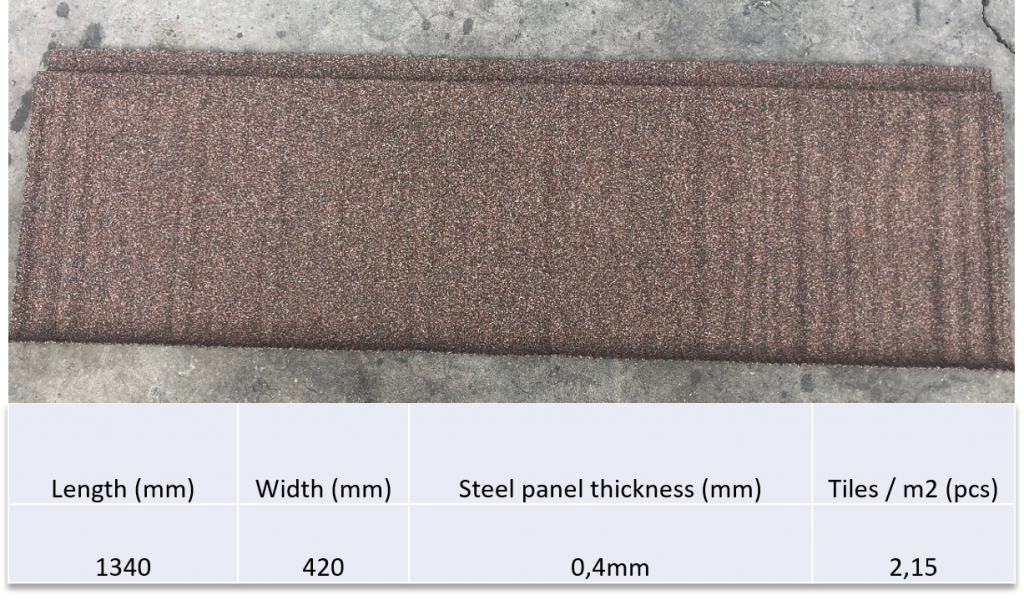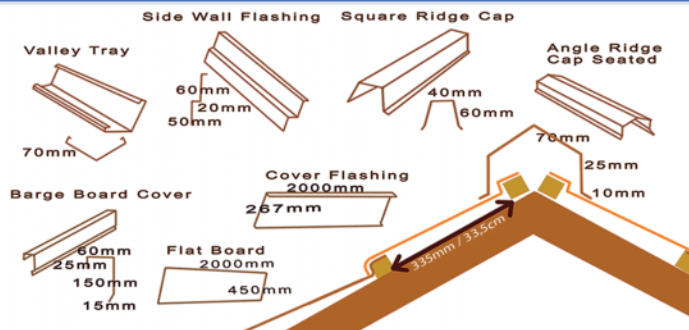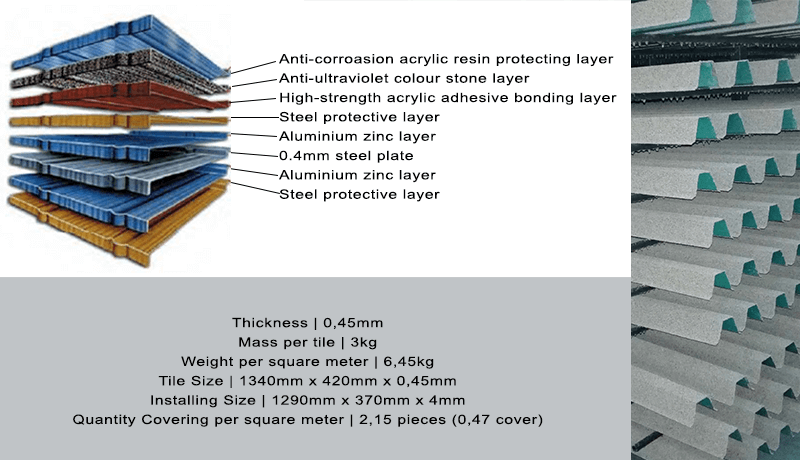Technical Info2019-08-05T12:00:24+00:00# FIDDLER™ TILE SIZE# ACCESSORIESAll accessories are 2m in length. All FIDDLER™ tiles come with a 30 year limited warranty# How to calculate the amount of roof tiles and accessories required for your roof project

## ROOF TILE CALCULATION

Example

Tile quantity calculation

If your roof square meter area is 100sqm

Each square meter requires 2,15 tiles

Take the roof sqm of 100 X 2,15 tiles = 215 tiles

The Theoretical number of tiles required are 215 tiles

Add a tile cutting loss of 10% to the 215 tiles to allow for cutting loss during installation

10% additional tiles to allow for cutting loss = 21,5 tiles

The total number of tiles required for a 100sqm  roof is: 215 + 21,5 = 236,5 tiles

You can thus order 237 tiles or rounded off to 240 tiles

## ACCESSORY CALCULATION

Example

Angle ridge cap calculation

Measure the total ridge lengths on the roof, eg. 60m

Each ridge cap length is 2 meter long

Take the 60m and devide it by 2m = 30

You will thus theoretically require 30 pieces of Angle ridges

Additional 10% = 3 Angle ridges

Your total number of Angle ridges required is:

30 + 3 = 33 Angle ridges

You can thus order 33 Angle ridges

The FIDDLER™ roof tiles require a minimum roof pitch of 12 degrees.